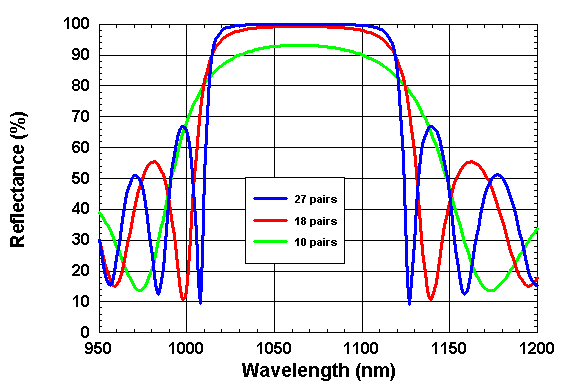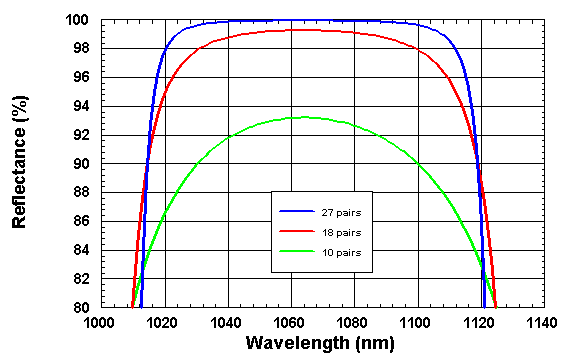> Energy band gap> GaAs | AlxGa1-xAs | InxGa1-xAs
> Refractive index > GaAs | AlAs | AlxGa1-xAs | InxGa1-xAs
> Devices > Bragg mirror | SAM | RSAM | SA | SANOS | SOC | Microchip | PCA
> Device application > Papers | Patents | FAQs## Bragg mirror

##### Construction of a Bragg mirror

A thin film Bragg reflector consists of a multilayer-stack of alternate high- and low-index films, all one quarter wavelength thick (see figure right). The geometrical thicknesses of the high- und low-index films are tH = λ/(4nH) and tL = λ/(4nL) respectively.
nH and nL are the indices of refraction of the high- and low-index films, respectively and λ is the center wavelength of the Bragg mirror.
On every interface in the stack a part of the incident beam is reflected. The reflected parts have a phase shift of 180° only if the incident light goes from low-index medium in a high-index medium. The relative phase difference of all reflected beams is zero or a multiple of 360° and therefore they interfere constructively.
The intensity of the incident light beam decreases during his travel trough the quarter-wave stack and at the same time the reflected light increases, if the absorptance A of the stack is negligible.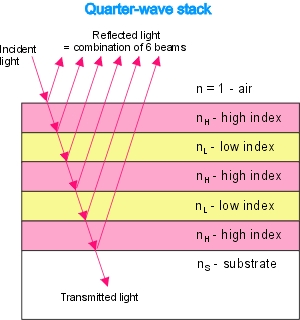Reflectance

Reflectance of a Bragg mirror

The reflectance R of a quarter-wave stack in air or free space with high-index layers outermost on both sides is given by :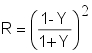with• Y - admittance of a quarter wave stack with (2p + 1) layers
• nH - high index of refraction
• nL -low index of refraction
• nS - index of the substrate
• (2p + 1) - number of layers in the stack

Approximation

If the number (2p + 1) of films in the quarter-wave stack is large and the absorption can be neglected then the reflectance R and the transmittance T can be approximated by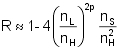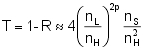Numeric examples

Reflectance of a Bragg mirror as a function of the number p of film pairs with nH = nS = 3.5 and nL = 3.0.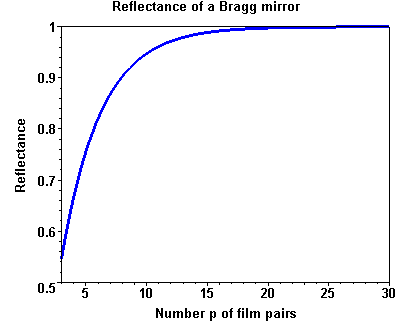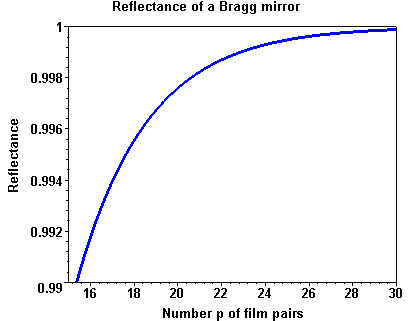Spectral width

Spectral width of the high refletion zone

The spectral width Δλ of the high reflectance zone increases with increasing difference of the refractive indices nH - nL.
Δλ can be estimated with the design wavelength λ0 of the quarter-wave stack by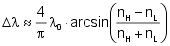The relative spectral width w = Δλ/λ of the high reflectance zone as a function of the ratio of the refractive indices nH/nL is shown in the figure right.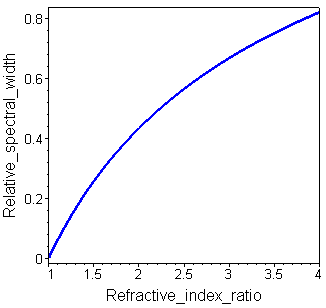AlAs/GaAs Bragg mirror

AlAs/GaAs Bragg mirror

For the wavelength 1064 nm the refractive indices of the materials GaAs and AlAs are n(GaAs) = 3.49 and n(AlAs) = 2.95, respectively.
The figures below show the spectral reflactance of AlAs/GaAS quarter-wave stacks with the center wavelength λ0 = 1064 nm and different numbers of AlAs/GaAs pairs.